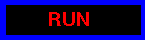OLIVER'S TWIST PAGES MAINPAGE -> ENTROPY DOWNLOAD JAVA APPLET SHOWROOM TWISTIE SLIDES LINKS 1999 © dynamical-systems.org
Entropy measurements
 You can numerically determine here, where T is the Chirikov Standard map T(x,y) = (2x-y+k sin(x),x). The sequence f(n) converges in the limit n to infinity to the Kolmogorov-Sinai entropy of the map (Pesin formula). The conjecture is thatfor all k and all n>0. (m determines the accuracy of the Riemann sum approximating the integral over the phase space. The program sums over a (m x m)-grid in the phase space.) Every 5 runs, your experiment results are submitted to our database. The computation done on your computer will so help to test the hypothesis. This is also an experiment in distributed computing.
 k:
 n:
 m:Experiments done Lower bound log(k/2) Last measurement Averaged